DC-DC Converter or Chopper

# DC-DC Converter or Chopper - Notes | Study Power Electronics - Electrical Engineering (EE)

 Table of contentsIntroduction1 Crore+ students have signed up on EduRev. Have you?

Introduction

A Chopper or DC‐to‐DC Converter is a static device which is used to obtain a variable variable dc voltage voltage from a constant constant dc voltage voltage source.

• The thyristor converter offers greater efficiency, faster response, lower maintenance, smaller size and smooth control control. Choppers Choppers are widely used in trolley trolley cars, battery operated vehicles, traction motor control, control of large number of dc motors, etc.
• They are also used in regenerative braking of dc motors to return energy back to supply and also as dc voltage voltage regulators regulators.• Power conversion deals with the process of converting electric power from one form to another.
• The power electronic devices performing the power conversion are called power converters.
• The power conversion is achieved using power semiconductor devices, which are used as switches.
• The power devices used are SCRs (silicon controlled rectifiers, or thyristors), triacs, power transistors, power MOSFETs, insulated gate bipolar transistors (IGBTs), and MCTs (MOS-controlled thyristors).

DC to DC Conversion

• DC-dc converters are used to convert unregulated dc voltage to regulated or variable dc voltage at the output.
• They are widely used in switch-mode dc power supplies and in dc motor drive applications.
• In dc motor control applications, they are called chopper-controlled drives.
• The input voltage source is usually a battery or derived from an ac power supply using a diode bridge rectifier.

Choppers are of two types

• Step‐down choppers
• Step‐up choppers

Note: In step‐down choppers, the output voltage will be less than the input voltage whereas in step‐up choppers output voltage will be more than the input voltage.

1. Principle of Step Down Chopper

• Figure shows a step‐down chopper with resistive load.
• The thyristor in the circuit acts as a switch.
• When thyristor is ON,supply voltage appears across the load and when thyristor is OFF, the voltage across the load will be zero.
• It is also called Buck- Converter.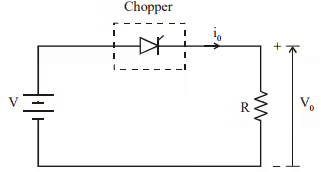Output Voltage Waveform of Step Down Chopper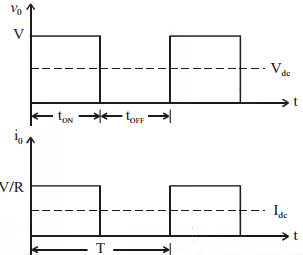Where

• Vdc = Average value of Output or Load Voltage.
• Idc = Average value of output  or Load Current.
• TON = Time interval for which SCR IS ON.
• TOFF = Time interval for which SCR is OFF.
• T = TON + TOFF = Period of Switching or Chopping Period.
• f = Frequency of Chopper Switching or Chopping Frequency.
• D = TON/T

Average Output Voltage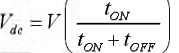Vdc = V(Ton/T) = V.D
Average output current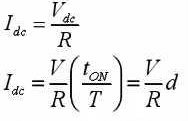RMS value of output voltage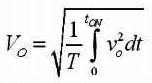But during tON, v0 = V
Therefore RMS output voltage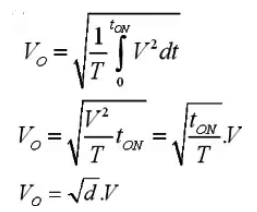Output power
Po = VoIo
But Io = Vo/R
Therefore output power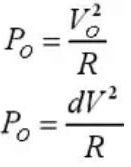Effective input resistance of chopper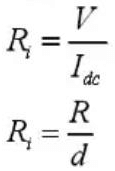The Output Voltage can be varied by varying the output voltage.

2. Principle of Step-Up Chopper

• This converter is used to produce higher voltage at the load than the supply voltage.
• When the power switch is on, the inductor is connected to the dc source and the energy from the supply is stored in it.
• When the device is off, the inductor current is forced to flow through the diode and the load.
• The induced voltage across the inductor is negative.
• The inductor adds to the source voltage to force the inductor current into the load.
• It is also called as Boost Converter.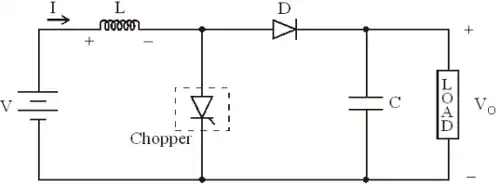• The output voltage is given by: Vout = Vin / (1 – D)
• Thus for variation of D in the range 0 < D < 1, the load voltage Vout will vary in the range Vin < Vout < ∞.

Expression for Output Voltage
Assume the average inductor current to be I during OS and OFF time of Chopper

1. When Chopper is ON
Voltage across inductor L = V
Therefore energy stored in inductor = V .I.tON
where tON = ON penod of chopper.
2. When Chopper is OFF (energy is supplied by inductor to load)
Voltage across L = V0 - V
Energy supplied by inductor L = (V0 - V)ItOFF, where tOFF = OFF period of Chopper.
Neglecting losses, energy stored in inductor Z = energy supplied by inductor L
There fore VItON = (Vo - V)ItOFF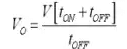Buck-Boost Converter

• A buck-boost converter can be obtained by the cascade connection of the buck and the boost converter which is shown in the following figure.
• When the power device is turned on, the input provides energy to the inductor and the diode is reverse biased.
• When the device is turned off, the energy stored in the inductor is transferred to the output.
• No energy is supplied by the input during this interval.
• In dc power supplies, the output capacitor is assumed to be very large, which results in a constant output voltage.
• In dc drive systems, the chopper is operated in step-down mode during motoring and in step-up mode during regeneration operation.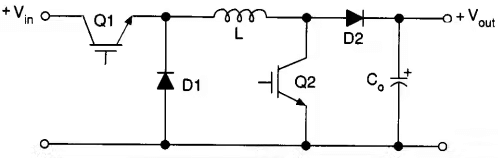The steady-state output voltage Vout is given by: Vout = Vin D / (1 – D)
This allows the output voltage to be higher or lower than the input voltage, based on the duty cycle D.

The use of resonant converter topologies would help to reduce the switching losses in dc-dc converters and enable the operation at switching frequencies in the megahertz range.
By operating at high frequencies, the size of the power supplies could be reduced.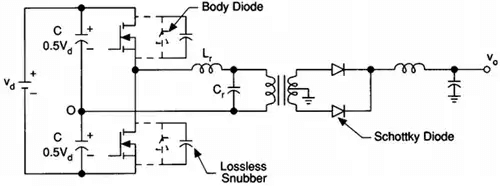• The dc power is converted to high-frequency alternating power using the MOSFET half-bridge inverter.
• The resonant capacitor voltage is transformer-coupled, rectified using the two Schottky diodes, and then filtered to get output dc voltage.
• The output voltage is regulated by control of the inverter switching frequency.
• Instead of parallel loading as in the above figure, the resonant circuit can be series-loaded; that is, the transformer in the output circuit can be placed in series with the tuned circuit.
• The series resonant circuit provides short-circuit limiting feature.
The document DC-DC Converter or Chopper - Notes | Study Power Electronics - Electrical Engineering (EE) is a part of the Electrical Engineering (EE) Course Power Electronics.
All you need of Electrical Engineering (EE) at this link: Electrical Engineering (EE)

## Power Electronics

5 videos|39 docs|63 tests
 Use Code STAYHOME200 and get INR 200 additional OFF

## Power Electronics

5 videos|39 docs|63 tests

Track your progress, build streaks, highlight & save important lessons and more!

,

,

,

,

,

,

,

,

,

,

,

,

,

,

,

,

,

,

,

,

,

;